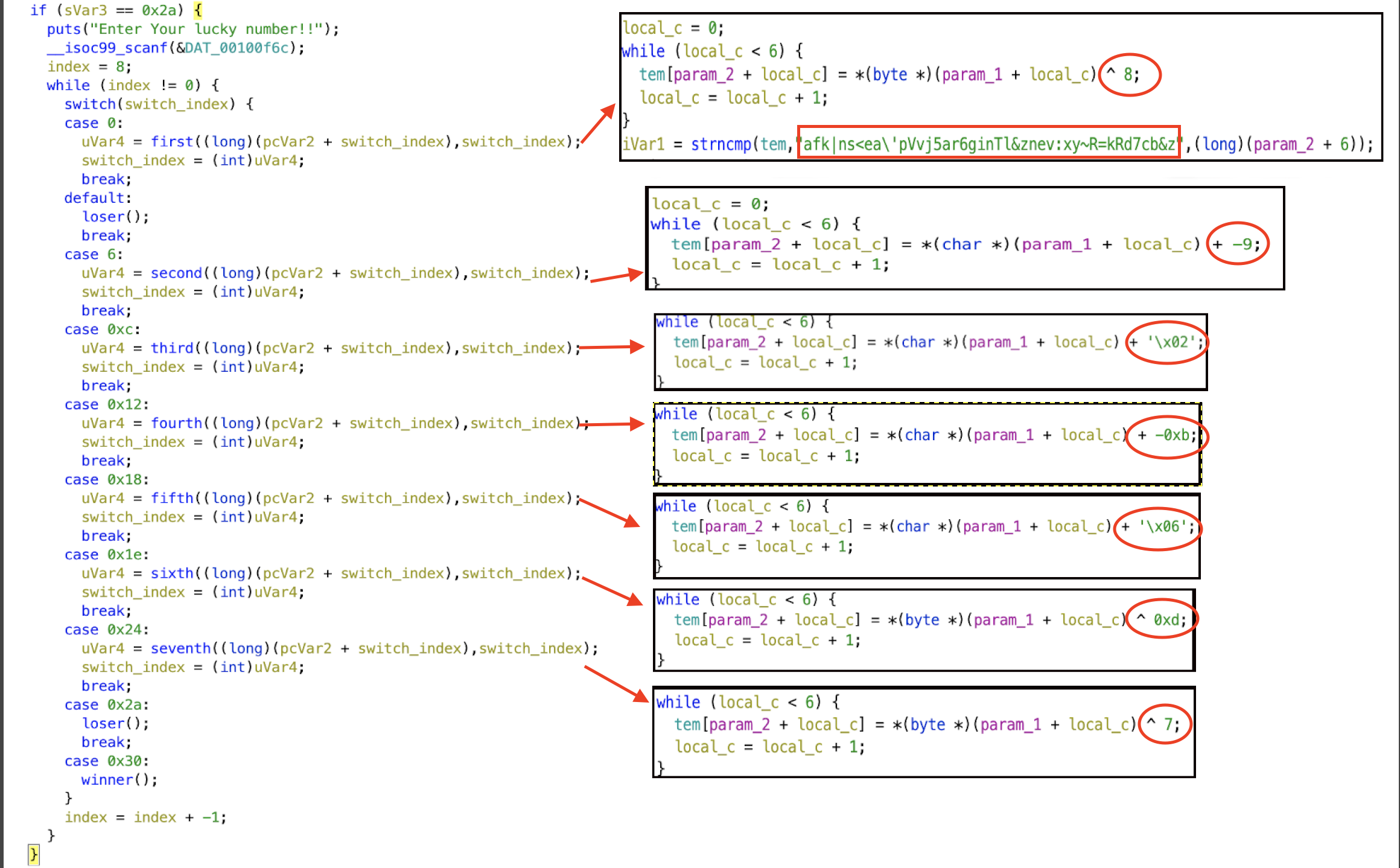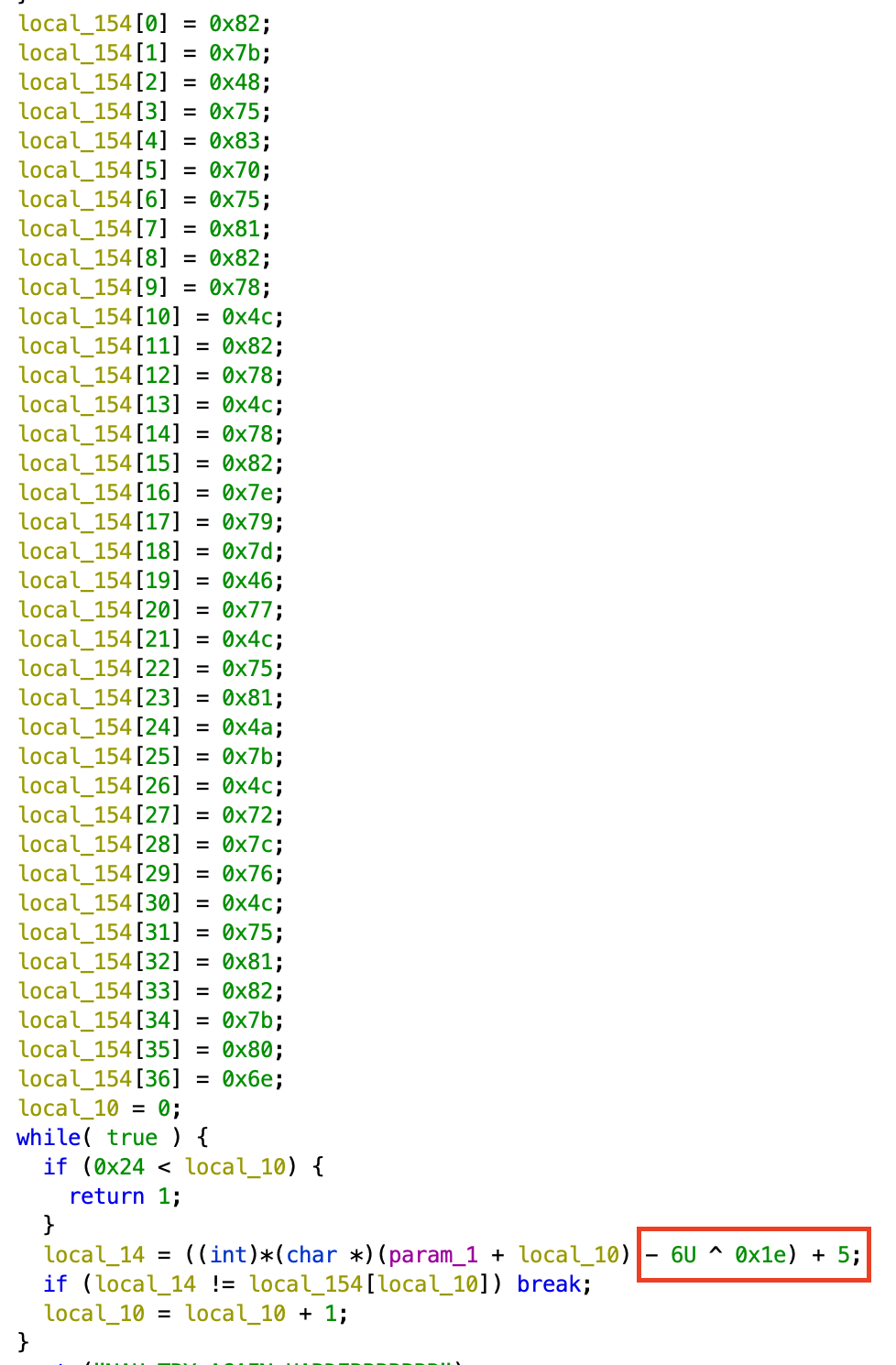# inCTF 2019 Finals writeup

Writeup for inctf professional that i solved

## Forensics - The Dilema

This is the first challenge i done it is pretty simple

you have given a zip file which is password protected you need to crack it which i did it using fcrackzip ( Dictionary attack - rockyou as wordlist ).

after extracting we get

• inCTF-Final.jpg
• text.txt

YodaMaster

I tried stegno decoder with above text as password, got my first flag.

https://futureboy.us/stegano/decinput.html used this to decode message.

``inctf{w0w_D0_Y0u_w4Nt_Th3_F0Rc3_t0_b3_w1Th_Y0u}``

## Reverse Engineering - Party part

The binary is elf-64. when we load in ghidra (NSA reverse engineering tool) we can see it like this• you can see the red rectangle is the flag in encrypted format each function decode it by 6 character and increment the pointer to the next 6 repeat until flag end.
• Red circle in the diagram represents operation done in each function to encrypt the flag.

using the above information, it can be decoded.i used python3 to decode the all the function

NOTE: \’ in the encrypted flag treated as ’ ( single character )

``````first_encrypt = "afk|ns"
#inctf{
for char in first_encrypt:
ascii_number = ord(char)
manipulated = ascii_number^8
print(chr(manipulated))

second_encrypt = "<ea'pV"

for char in second_encrypt:
ascii_number = ord(char)
manipulated = ascii_number+9
print(chr(manipulated))

thrid_encrypt = "vj5ar6"

for char in thrid_encrypt:
ascii_number = ord(char)
manipulated = ascii_number-2
print(chr(manipulated))

four_encrypt = "ginTl&"
for char in four_encrypt:
ascii_number = ord(char)
manipulated = ascii_number+11
print(chr(manipulated))

five_encrypt = "znev:x"
for char in five_encrypt:
ascii_number = ord(char)
manipulated = ascii_number-6
print(chr(manipulated))

six_encrypt="y~R=kR"
for char in six_encrypt:
ascii_number = ord(char)
manipulated = ascii_number^13
print(chr(manipulated))

seven_encrypt = "d7cb&z"
for char in seven_encrypt:
ascii_number = ord(char)
manipulated = ascii_number^7
print(chr(manipulated))

#encrypted="afk|ns<ea\ 'p Vvj5ar6 ginTl& znev:x y~R=kR d7cb&z"
``````

and when you run it we got

``````
inctf{Enj0y_th3_p4rty_w1th_p4rts_0f_c0de!}
``````

## Web - Php

I don’t remember the challenge exactly so i will give you picture of how it will be

It is php page we need to bypass the if statement to print the flag.All the input are given as GET parameters.

There are four if we need to bypass inorder to get the flag

1. number which is greater than 999999999 ( forgot the exact number )
2. int(given parameter must be 1337) && it should be 13 character
3. SHA-4 collision ( Two param using a & b )
4. MD-4 => (\$_GET[“md4”] == hash(“md4”, \$_GET[“md4”]))

Solving each part you get part of the flag

First two are pretty easy. the challenge get complicated from 3

I solved Third using the below link

https://github.com/bl4de/ctf/blob/master/2017/BostonKeyParty_2017/Prudentialv2/Prudentialv2_Cloud_50.md

and fourth by

https://medium.com/@sbasu7241/hsctf-6-ctf-writeups-a807f0b25ae4

After you beat all four if statement, got the flag

``````
inctf{PHP_H45_M4NY_WAYS_TO_BE_EXPLOITED!!}
``````

## Android - Decode

Simplest challenge of all if you know how to decode the .apk file and my first android challenge that i solved.

i used apktool tool to extract the contents of the .apk and it also generates smali files which we can further used to analyze the android package

``````    .line 20
new-instance p1, Ljava/lang/StringBuilder;

invoke-direct {p1}, Ljava/lang/StringBuilder;-><init>()V

const-string v0, "aW5jdGZ7bmV2M3Jfc3QwcmU="

invoke-virtual {p1, v0}, Ljava/lang/StringBuilder;->append(Ljava/lang/String;)Ljava/lang/StringBuilder;

const-string v0, "X3MzbnMhdGl2M19kYXRh"

invoke-virtual {p1, v0}, Ljava/lang/StringBuilder;->append(Ljava/lang/String;)Ljava/lang/StringBuilder;

const-string v0, "XzFuXzdoM19zMHVyY2VjMGRlfQ=="
``````

Bizzare strings in the above code is base-64 so decoded it ( decode all three ) and got the flag

``inctf{nev3r_st0re_s3ns!tiv3_data_1n_7h3_s0urcec0de}``

## TRIED

### Binary - shell

I got 32 bit binary which execute anything that you gave

so i copied shellcode from shellstorm x86 ( 32 bit ) and tried to run it and it didn’t work i wasted maybe an hour on that i tried using gdb to find issue but still can’t figure it out..

last few bytes of my payload got truncated i don’t know why :(

### Forensics - Moz Espa

you got a pcap file if you follow the Tcp stream you get the flow of how the person got interacted with the server

using that information you need to type the character one by one along with authentication ( random number ) before certain time inorder to get the flag

## Solved at home

### Reverse Engineering -

windows binary name fin.exe is givenAbove image you can see the operation ** -6U ^ 0x1e) + 5 ** which is used to encode the flag

solved the challenge using following python3 code

NOTE: converted 0x1e (hex) to 30 (decimal) in below code

``````input_array=[0x82,0x7b,0x48,0x75,0x83,0x70,0x75,0x81,0x82,0x78,0x4c,0x82,0x78,0x4c,0x78,0x82,0x7e,0x79,0x7d,0x46,0x77,0x4c,0x75,0x81,0x4a,0x7b,0x4c,0x72,0x7c,0x76,0x4c,0x75,0x81,0x82,0x7b,0x80,0x6e]

for element in input_array:
decoded = ((element - 5) ^ 30) + 6
print(chr(decoded),end='')
``````

flag is

``````
inctf{this_is_simpler_than_you_think}
``````